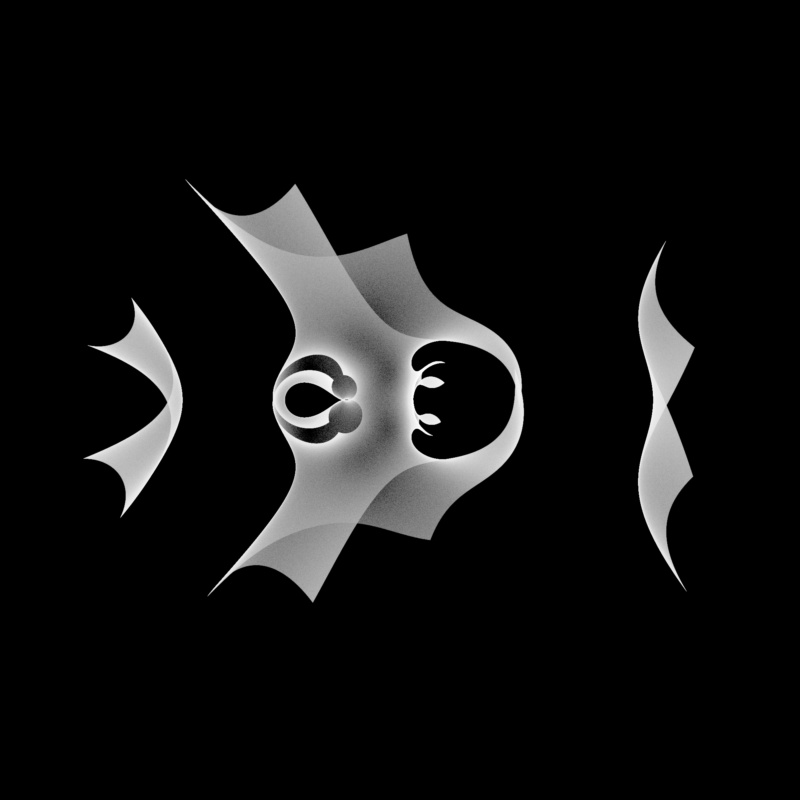# Bohemian MatricesEigenvalues of the matrix $$\begin{bmatrix} 1 & 1 & 1 & A & 0 & 0\\ 0 & 1 & 1 & 0 & 0 & 0\\ 1 & 1 & 0 & 1 & 0 & 1\\ 1 & 0 & 1 & 0 & B & 0\\ 0 & 0 & 1 & 0 & 0 & 0\\ 0 & 0 & 1 & 0 & 1 & 0 \end{bmatrix}$$ where $X$ is a continuous uniform random variable on $(0, 1)$, and $A$ and $B$ are independently sampled from $9Xi - 5i$. This image represents a sample of 5 million matrices. Note that this class of matrices is not Bohemian as it is sampled from a continuous distribution. Viewed on [-3-3.5i, 4+3.5i]. Real eigenvalues have been omitted from this plot.